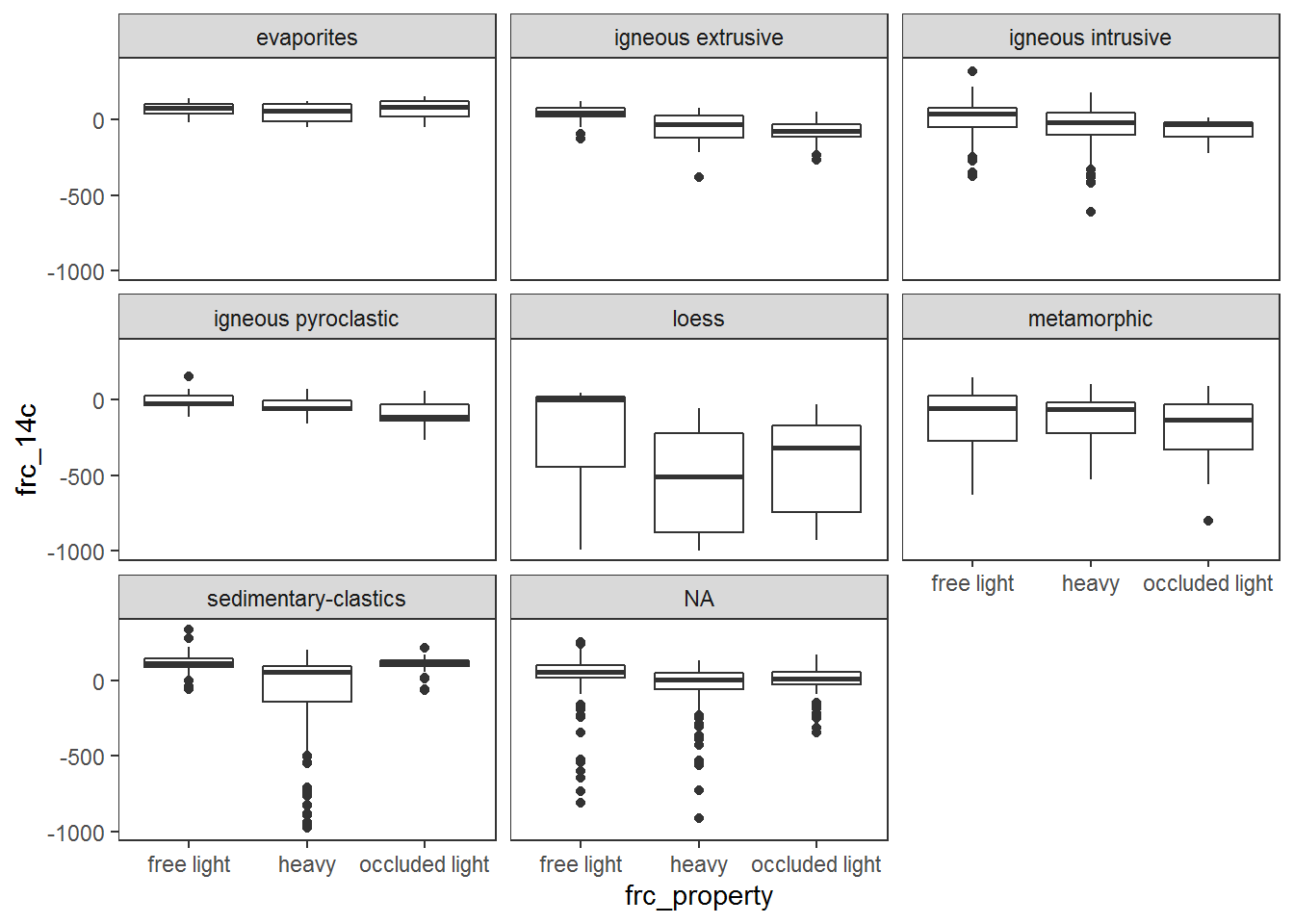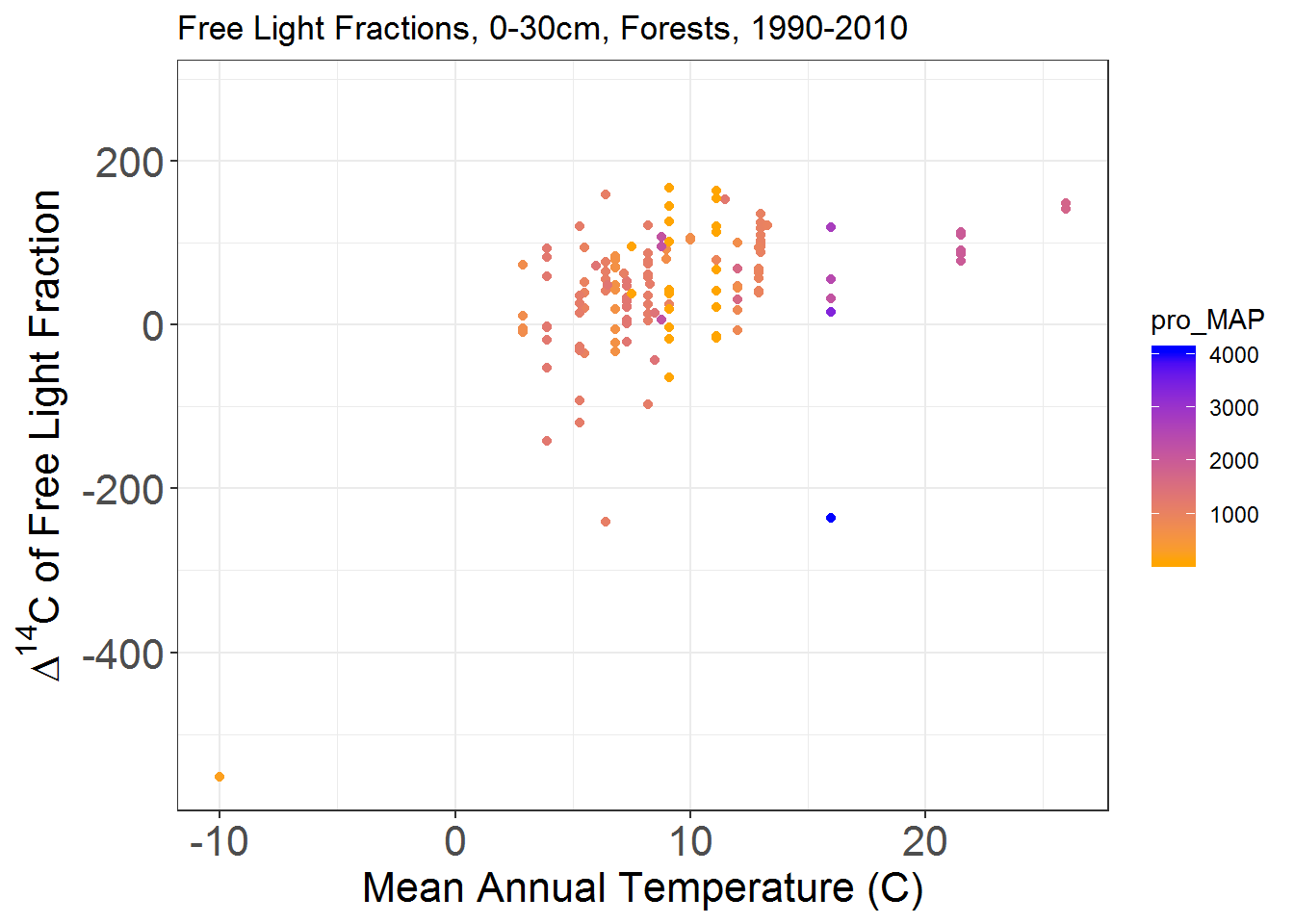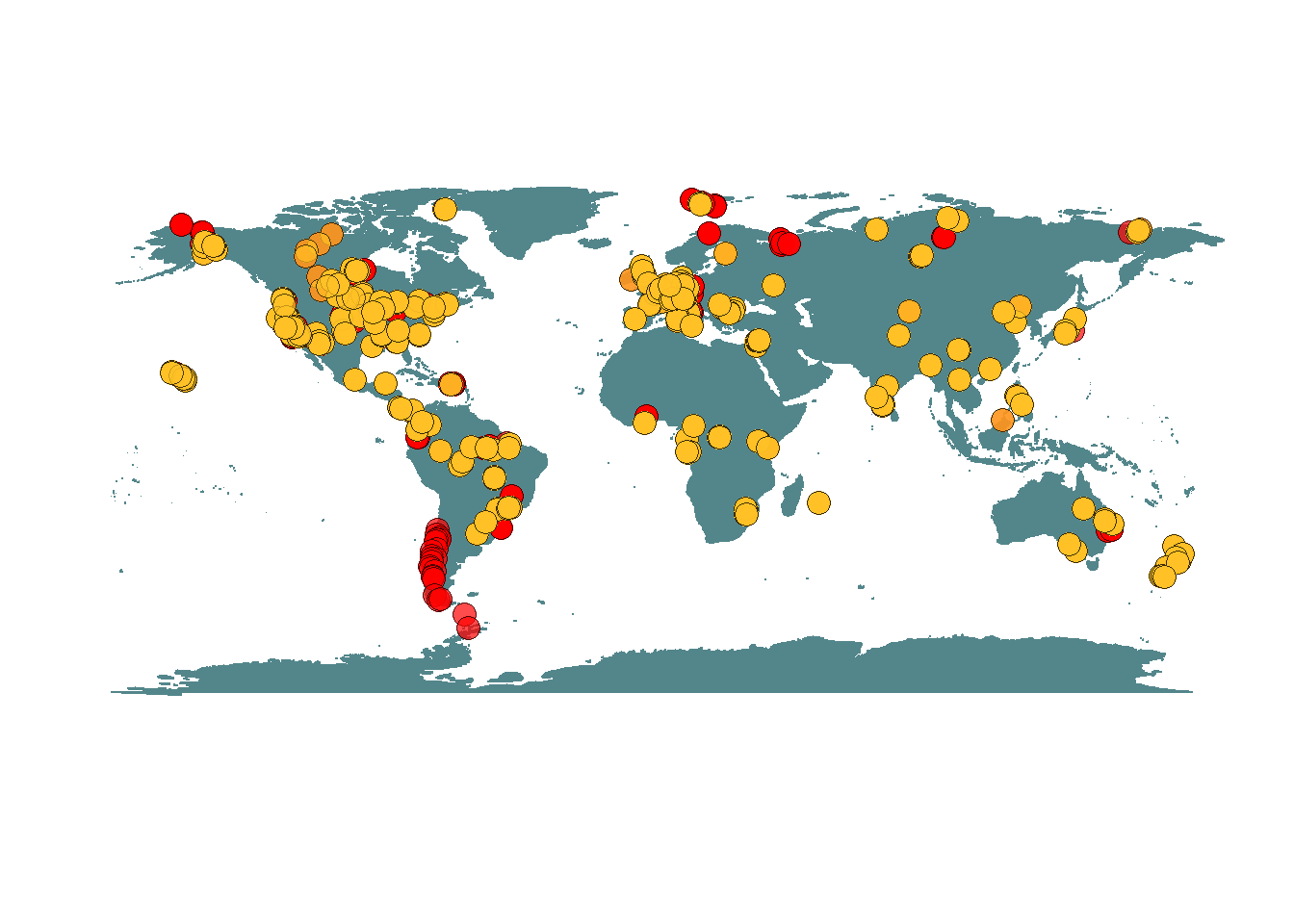### Helpful packages for this tutorial:

#Packages to install (you only need to do this once)
#For data analysis:
install.packages("tidyverse") #includes dplyr, ggplot2
install.packages("maps")
install.packages("ggmap")

install.packages("devtools")
install.packages("rcrossref")

#Load these packages (you need to do this every time you restart R)
library(tidyverse)
library(maps)
library(ggmap)
library(devtools)
library(rcrossref)

## Working with the database in R

1) ISRaD_data: The complete collection of data, reported from publications, compiled in one place
2) ISRaD_extra: An augmented dataset, with useful global variables and radiocarbon calculations completed for you
3) Tools: Options to compile your own dataset to compare to ISRaD, and functions to work with the data

2. Unzip the files
3. Load the .rda files into R. Specify the folder.
load("C:/Users/YourPathHere/ISRaD_data_v1-2019-08-13.rda") #Loads ISRaD_data
load("C:/Users/YourPathHere/ISRaD_data_v1-2019-08-13.rda") #Loads ISRaD_extra

#### Install the data through the ISRaD R package:

1. Install and load the ‘devtools’ and ‘rcrossref’ packages
2. Install the ISRaD package from Github
# 'devtools' allows you to install the ISRaD package in its "beta"/development form
# 'rcrossref' is used by our QAQC tool, but sometimes ISRaD won't install without it
install.packages("devtools")
library(devtools)
install.packages("rcrossref")
library("rcrossref")
# 2) Install package 'ISRaD' from the development branch of our github repository:
library(ISRaD) # load the package
# 3) Load data using the get_data function:
mydir <- "C:/Users/YourPathHere/"
ISRaD_extra <-ISRaD.getdata(directory = mydir, dataset="full", extra = T, force_download = T)

#### Some other useful packages for working with the data:

Tidyverse and dplyr (part of tidyverse) are useful packages for exploration of ISRaD data. This is a helpful cheatsheet.

We use packages dplyr for filtering data and ggplot for plotting. Both packages are included in the tidyverse library.

install.packages("tidyverse")
library(tidyverse)

install.packages("ggplot2") #should be included in tidyverse, but you may want to install separately
library(ggplot2)

The ISRaD data are formated as a list in an R object called ISRaD_data. ISRaD_data is a list comprised of 8 data.frames (metadata, site, profile, flux, layer, interstitial, fraction, and incubation), which correspond to the template data tables. ‘ISRaD_extra’ follows the same structure. Here is a conceptual overview of the data structure.

#Check to make sure the package is loaded by looking for the database object "ISRaD_data"
#View a single table (e.g. Site, Profile, Layer, etc):
#This will show you all the sites compiled in the database
View(ISRaD_data$Site) ## Creating Your Own “Flat” Data Frame of Interest Ready to start your analysis? You may want to create your own “flat” data frame with the data of interest to you. To do this you can: 1. Use the ISRaD built-in flatten function and specify the table of interest. The function flatten creates a data frame with all relevant layers of the hierarchy. You can create a flat data frame with data from the flux, layer, interstitial, fraction or incubation tables. You can flatten data from ISRaD_data, ISRaD_extra, or your own compiled data with a similar structure. inc_data <- ISRaD.flatten(ISRaD_extra, 'incubation') lyr_data <- ISRaD.flatten(ISRaD_extra, 'layer') frc_data <- ISRaD.flatten(ISRaD_extra, 'fraction') 1. Or flatten the data yourself by joining the data of interest with other levels of the hierarchy. This does the same thing! #Flatten layer data: lyr_data <- ISRaD_extra$layer %>% #Start with layer data
left_join(ISRaD_extra$profile) %>% #Join to profile data left_join(ISRaD_extra$site) %>% #Join to site data
left_join(ISRaD_extra$metadata) #Join to metadata #or merge fraction data with other data up the hierarchy in an object called "frc_data" frc_data <- ISRaD_extra$fraction %>% #Start with fraction data
left_join(ISRaD_extra$layer) %>% #Join to layer data left_join(ISRaD_extra$profile) %>% #Join to profile data
left_join(ISRaD_extra$site) %>% #Join to site data left_join(ISRaD_extra$metadata)

#Take a look at it:
View(frc_data)

inc_data <- ISRaD_extra$incubation %>% #Start with incubation data left_join(ISRaD_extra$layer) %>% #Join to layer data
left_join(ISRaD_extra$profile) %>% #Join to profile data left_join(ISRaD_extra$site) %>% #Join to site data
left_join(ISRaD_extra$metadata) Or join with a more limited set of information: #Merge flux data with site information only flx_data <- ISRaD_data$flux %>% #Start with flux data

#Take a look at it:
View(flx_data)

## Filtering the Data & Summary Statistics

#### How much incubation data do we have? How is it distributed? To answer this:

2. Filter by depths less than 50cm
3. Group by land cover class
4. Print the number of data points and the mean 14C for each class
inc_data %>%
filter(lyr_bot < 50) %>% #filters depths above 50cm.
filter(is.na(inc_14c) != TRUE) %>% #filters 14C data only
group_by(pro_land_cover) %>% #groups by land cover class
summarise(num_data_points = n(), #summarizes number of points
mean_inc_14c = mean(inc_14c, na.rm=TRUE)) #Calculates mean 14C
## # A tibble: 8 x 3
##   pro_land_cover      num_data_points mean_inc_14c
##   <fct>                         <int>        <dbl>
## 1 bare                              8         49.1
## 2 cultivated                       56        119.
## 3 forest                          623         93.7
## 4 rangeland/grassland              36         46.9
## 5 shrubland                        38         30.1
## 6 tundra                          223         16.6
## 7 wetland                          78         62.1
## 8 <NA>                            156        -40.4

## Filter & Plot:

#### How do the density fractions in forests compare?

frc_data %>%
filter(frc_scheme == "Density") %>%
filter(frc_property != "NA") %>%
ggplot()+
geom_boxplot(aes(x=frc_property, y = frc_14c)) +
theme_bw()+theme(panel.grid.major = element_blank(), panel.grid.minor = element_blank())+
facet_wrap(~pro_parent_material) #Makes one plot for each parent material#### Is there any temperature dependence in the free light fraction?

frc_data %>%
dplyr::filter(lyr_bot <= 30 & lyr_top >=0) %>%
dplyr::filter(pro_treatment == 'control') %>%
dplyr::filter(pro_land_cover == "forest") %>%
dplyr::filter(frc_scheme == "Density") %>%
dplyr::filter(frc_property == "free light")%>%
dplyr::filter(lyr_obs_date_y >=1990 & lyr_obs_date_y <= 2010) %>%
dplyr::filter(is.na(frc_14c) != TRUE) %>%
#mutate(bin_temp2=cut(pro_MAT_wc, 4)) %>% #Add 4 temperature bins
ggplot()+
geom_point(aes(x = pro_MAT, y= frc_14c, color = pro_MAP))+
theme_bw()+
xlab("Mean Annual Temperature (C)")+
ylab(expression(Delta^14 *"C of Free Light Fraction")) +
ggtitle("Free Light Fractions, 0-30cm, Forests, 1990-2010")+
scale_color_gradient(low = "orange", high = "blue")+
theme(axis.text=element_text(size=16),
axis.title=element_text(size=16))#### Map all ISRaD layer data, and sites with 14C layer data

install.packages("maps")
install.packages("ggmap")
library(maps)
library(ggmap)

First setup the basemap:

#Maps:
world_map <- map_data("world")

#Creat a base plot with gpplot2
p <- ggplot() + coord_fixed() +
xlab("") + ylab("")

#Pick color words here: http://sape.inf.usi.ch/quick-reference/ggplot2/colour
base_world_messy <- p + geom_polygon(data=world_map, aes(x=long, y=lat, group=group),

cleanup <- theme(panel.grid.major = element_blank(), panel.grid.minor = element_blank(),
panel.background = element_rect(fill = 'white', colour = 'white'),
axis.line = element_line(colour = "white"), legend.position="none",
axis.ticks=element_blank(), axis.text.x=element_blank(),
axis.text.y=element_blank())

base_world <- base_world_messy + cleanup
#base_world #Plots map to screen

Plot locations of ISRaD profiles on the map. Yellow points have bulk soil (layer) 14C data. Red points have other data, but do not have bulk soil 14C data.

#Filter only data with 14C:
lyr_data_14c <- lyr_data %>%
dplyr::filter(is.na(lyr_14c) != TRUE)

map_data <-
base_world +
geom_point(data=lyr_data, #lyr_all, #put incubation data here to plot points
aes(x=pro_long, y=pro_lat), colour="black",
shape = 21, size=4, alpha=I(0.7), fill = "red")+
geom_point(data=lyr_data_14c, #lyr_all, #put incubation data here to plot points
aes(x=pro_long, y=pro_lat), colour="black",
shape = 21, size=4, alpha=I(0.7), fill = "goldenrod1")#+

map_data # Plots map to screen## Compiling your own data offline:

Advanced users may wish to compile thier own data locally in order view it in the context of the larger database. (note: that this operation is not the same as submitting your data for ingest)

The compile function is used QA/QC and assemble additional datasets (that pass the QA/QC test) into an new list, which can later be merged with ISRaD_data.

In order to run compile on a set of user specified data entries, the user must create a local folder whose path is specified with dataset_directory. This folder must only contain the entries to be compiled in .xlsx format. If other files types exist in the directory, compile will fail. (note: entries cannot be open in Excel)

compiled<-compile(dataset_directory = "~/Directory/to/data/", write_report = T, write_out = T, return="list")

The paramter return determines format of the object that is returned and should be set to “list” unless the user prefers a flattend version of the database formatted as a single data.frame.

When set to “TRUE”, the parameter write_out will trigger the creation of several output files:

Description Location File name
Report files that identify issues with the files in the dataset_directory dataset_directory/QAQC QAQC_*.txt (* corresponds to dataset file names)
### Merging a user compiled list with ISRaD_data
The function mapply can be used to merge the user compiled list with ISRaD_data as follows:
merged_data<-mapply(rbind, ISRaD_data, compiled, SIMPLIFY=FALSE)